What's new
Fantasy Football - Footballguys Forums

Welcome to Our Forums. Once you've registered and logged in, you're primed to talk football, among other topics, with the sharpest and most experienced fantasy players on the internet.

# Daughter's math homework (1 Viewer)

#### MrPack

##### reformed delinquent
Maybe I'm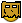but here goe.......

Solve this problem:

-5² + 4 x 2³ =

-6² + 2 x 3² =

Last edited by a moderator:
1st one = 72nd one = -18

Maybe I'mbut here goe.......

Solve this problem:

-5² + 4 x 2³ =

-6² + 2 x 3² =

1. 572. 54

Order of operations. First resolve the exponents, then the multiplication, then the addition.

Last edited by a moderator:
1st one = 7

2nd one = -18
Sonova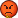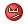Last edited by a moderator:
1st one = 7

2nd one = -18
depends if you look at as -(5^2) or (-5)^2. I assume the latter, but could be wrong.

Maybe I'mbut here goe.......

Solve this problem:

-5² + 4 x 2³ =

-6² + 2 x 3² =

1. 572. 54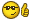Too bad the teacher sees it bryhamms way

1st one = 7

2nd one = -18
depends if you look at as -(5^2) or (-5)^2. I assume the latter, but could be wrong.
I agree, but it's written exactly how I typed it, no parentheses

1st one = 7

2nd one = -18
depends if you look at as -(5^2) or (-5)^2. I assume the latter, but could be wrong.
No, I think bryhamm's right. Unless it's specifically (-5)^2 then "-5^2" is -25, not 25. I think the teacher is right.There was a thread about this before. I think Shick! cleared it all up.

Maybe I'mbut here goe.......

Solve this problem:

-5² + 4 x 2³ =

-6² + 2 x 3² =

no parentesis??? could be 1. 232 or 57 I think 57.. I was always taught mult/div takes precedence over add/sub

2. 342 or 54 I say 54

Last edited by a moderator:
#1 - 57#2 - 54A negative number raised to an even power makes a positive number. Raised to an odd power is a negative number.What's the teacher saying?

Maybe I'mbut here goe.......

Solve this problem:

-5² + 4 x 2³ =

-6² + 2 x 3² =

1. 572. 54

Order of operations. First resolve the exponents, then the multiplication, then the addition.
-5² + 4 x 2³ = 25 + 32 = 57

-6² + 2 x 3² = 36 + 18 = 54
You do the exponentials first, and since there is not a parenthesis around the 5 in the first one or the 6 in the 2nd one, you end up with -25 and -36 respectively.Had they wanted 25 and 36, then it should have been written (-5)² and (-6)²

1st one = 7

2nd one = -18
depends if you look at as -(5^2) or (-5)^2. I assume the latter, but could be wrong.
I agree, but it's written exactly how I typed it, no parentheses
Exactly. I think we're wrong. I think -5^2 is -25 using modern standards. To get 25 would require writing (-5)^2.

1) 57

2) 54

Parentheses

Exponents

Multiplication

Division

Subdtraction

you're done
This is exactly how my daughter worked it. The teacher marked them both wrong.

#1 - 57

#2 - 54

A negative number raised to an even power makes a positive number. Raised to an odd power is a negative number.

What's the teacher saying?
It's a positive number being raised to a power, and then negated. The minus sign is the same as multiplying by -1, and thus isn't done until the multiplication step in the order of operations.

1st one = 7

2nd one = -18
depends if you look at as -(5^2) or (-5)^2. I assume the latter, but could be wrong.
No, I think bryhamm's right. Unless it's specifically (-5)^2 then "-5^2" is -25, not 25. I think the teacher is right.There was a thread about this before. I think Shick! cleared it all up.
-5² should = -25 right? It's a negative x negative

1st one = 7

2nd one = -18
depends if you look at as -(5^2) or (-5)^2. I assume the latter, but could be wrong.
I agree, but it's written exactly how I typed it, no parentheses
Exactly. I think we're wrong. I think -5^2 is -25 using modern standards. To get 25 would require writing (-5)^2.
I agree. It is easier to understand if you write it as -1 X 5^2

maybe it's just me, but I read that aloud as "negative 5 squared"...since when do negative numbers HAVE to be surrounded by parentheses?

#1 - 57

#2 - 54

A negative number raised to an even power makes a positive number. Raised to an odd power is a negative number.

What's the teacher saying?
Teacher says 7 and -18

1) 57

2) 54

Parentheses

Exponents

Multiplication

Division

Subdtraction

you're done
This is exactly how my daughter worked it. The teacher marked them both wrong.
The teacher is right.(-5)^2 != -5^2 because the latter is -(5^2). Order of operations.

1st one = 7

2nd one = -18
depends if you look at as -(5^2) or (-5)^2. I assume the latter, but could be wrong.
No, I think bryhamm's right. Unless it's specifically (-5)^2 then "-5^2" is -25, not 25. I think the teacher is right.There was a thread about this before. I think Shick! cleared it all up.
-5² should = -25 right? It's a negative x negative
No. New math says to think of "-5" as "5 x (-1)". So when you see "-5^2", you're actually seeing "-1 x 5^2".I know that's different from how we did it, but it seems to be the way it's being done these days.

Maybe I'mbut here goe.......

Solve this problem:

-5² + 4 x 2³ =

-6² + 2 x 3² =

1. 572. 54

Order of operations. First resolve the exponents, then the multiplication, then the addition.
-5² + 4 x 2³ = 25 + 32 = 57

-6² + 2 x 3² = 36 + 18 = 54
You do the exponentials first, and since there is not a parenthesis around the 5 in the first one or the 6 in the 2nd one, you end up with -25 and -36 respectively.Had they wanted 25 and 36, then it should have been written (-5)² and (-6)²
WTF? I don't remember this rule...doesn't make sense. The "-" is not a stand-alone operator...it must be combined with the number to make any sense.You can't compute the number and leave the "-" out there by itself, then throw it back in later.

That's like saying 12^2 is 14 (drop off the 1, compute the 2, add the one back in).

The number being computed is -5, not 5.

maybe it's just me, but I read that aloud as "negative 5 squared"...since when do negative numbers HAVE to be surrounded by parentheses?
Yes, but that can still be read two ways. Negative...5 squared or negative 5...squared.

1st one = 7

2nd one = -18
depends if you look at as -(5^2) or (-5)^2. I assume the latter, but could be wrong.
No, I think bryhamm's right. Unless it's specifically (-5)^2 then "-5^2" is -25, not 25. I think the teacher is right.There was a thread about this before. I think Shick! cleared it all up.
-5² should = -25 right? It's a negative x negative
negative * negative = positivenegative * negative * negative = negative

maybe it's just me, but I read that aloud as "negative 5 squared"...since when do negative numbers HAVE to be surrounded by parentheses?
Yes, but that can still be read two ways. Negative...5 squared or negative 5...squared.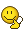negative 5, squared

WTF? I don't remember this rule.
No, it's new. They do it differently now. Shick! can explain it.

maybe it's just me, but I read that aloud as "negative 5 squared"...since when do negative numbers HAVE to be surrounded by parentheses?
Yes, but that can still be read two ways. Negative...5 squared or negative 5...squared.negative 5, squared
Right, but it's now being done such that the way it is written is to be read as negative, 5 squared.

1st one = 7

2nd one = -18
depends if you look at as -(5^2) or (-5)^2. I assume the latter, but could be wrong.
No, I think bryhamm's right. Unless it's specifically (-5)^2 then "-5^2" is -25, not 25. I think the teacher is right.There was a thread about this before. I think Shick! cleared it all up.
-5² should = -25 right? It's a negative x negative
No. New math says to think of "-5" as "5 x (-1)". So when you see "-5^2", you're actually seeing "-1 x 5^2".I know that's different from how we did it, but it seems to be the way it's being done these days.
Wow, I'll say it's different. And I used to be good in math.I will have to explain to her the bolded part. It certainly makes it a bit easier to understand.

WTF?  I don't remember this rule.
No, it's new. They do it differently now. Shick! can explain it.
Who the hell wrote this thesis to get this approved?The result the teacher is looking for used to be: -(5^2) + x - y....

It's an order of operations thing.(-5)^2 is said as "Negative five squared"-5^2 is said as "The negative of five squared" and as such, you square it first and then negate.

1st one = 7

2nd one = -18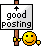-5² + 4 x 2³ =

-6² + 2 x 3² =

(1)

5 x 5 =25 x -1 = -25

2x2x2 = 8

4x8 = 32

-25 + 32 = 7

(2)

6x6 = 36 x -1 = -36

3x3 = 9

2x9 = 18

-36+18 = -18

1st one = 7

2nd one = -18
depends if you look at as -(5^2) or (-5)^2. I assume the latter, but could be wrong.
No, I think bryhamm's right. Unless it's specifically (-5)^2 then "-5^2" is -25, not 25. I think the teacher is right.There was a thread about this before. I think Shick! cleared it all up.
-5² should = -25 right? It's a negative x negative
negative * negative = positivenegative * negative * negative = negative
All this negativity is making me depressed... I'm out

It's an order of operations thing.

(-5)^2 is said as "Negative five squared"

-5^2 is said as "The negative of five squared" and as such, you square it first and then negate.
Yes. We've established that that's the right answer today. Do you remember, though, that that was not taught that way back when we were in school?

It's basically done out of necessity.You can write something 3 ways:-5^2(-5)^2-(5)^2For clarities sake, -5^2 is defined as -(5)^2, it's just a rule and it's been that way since I was little.

I put these equations in a scientific calculator and still came up with 75 and 54. -5² came out as 25, not -25.This is frigging nuts

To get 7 &amp; 18 as answers, the equations should have been written:-(5^2) + 4 X 2^3and-(6^2) + 2 X 3^2At least this is what I recall from my math classes. Why change something like this for "new" math?

It's basically done out of necessity.

You can write something 3 ways:

-5^2

(-5)^2

-(5)^2

For clarities sake, -5^2 is defined as -(5)^2, it's just a rule and it's been that way since I was little.
It was most definitely not that way when I went to school. How old are you?

It's an order of operations thing.

(-5)^2 is said as "Negative five squared"

-5^2 is said as "The negative of five squared" and as such, you square it first and then negate.
Yes. We've established that that's the right answer today. Do you remember, though, that that was not taught that way back when we were in school?
It's been like that as far back as I can remember. Course, I was taught this stuff in the 80's...dunno how far back y'all go. It's just one of those cooky definitions you have to have drilled in your head, that makes little logical sense but is necessary later on in math, like the order of operations.

It's basically done out of necessity.

You can write something 3 ways:

-5^2

(-5)^2

-(5)^2

For clarities sake, -5^2 is defined as -(5)^2, it's just a rule and it's been that way since I was little.
It was most definitely not that way when I went to school. How old are you?I was taught like this:

Brewdude Posted Today, 05:45 PM

To get 7 &amp; 18 as answers, the equations should have been written:

-(5^2) + 4 X 2^3

and

-(6^2) + 2 X 3^2

At least this is what I recall from my math classes. Why change something like this for "new" math?

if they want a negative square they should have written the question as-(5^2)which would be -25if you write -5^2 = -5 x -5 = +25the teacher is motarded

So MrPack, Bewdude and I were all taught the same way, and we're decidedly not from the same geographic area. So I don't know where roly went to school, but he's either much younger, or his district was way ahead of its time.

Yeah, as someone said earlier I think they take the negative sign as a multiplicaiton of (-1) times a number. So:-5^2 really is (-1)x(5)^2 whereas (-5)^2 is (-1x5)^2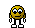This is why I hate double negatives.

Yeah, as someone said earlier I think they take the negative sign as a multiplicaiton of (-1) times a number. So:

-5^2 really is (-1)x(5)^2 whereas (-5)^2 is (-1x5)^2I was taught to do -5^2 as 25 because of no parethesis. If parenthesis then it would be negative because the negative is outside the rules of doing exponents first. I was taught in the mid 90's though. Dont know if I am in your group or rolyaTy's.

IIRC, I was taught this stuff in the early to mid 60s. The way I was taught was that -5^2 == -25

IIRC, I was taught this stuff in the early to mid 60s. The way I was taught was that -5^2 == -25
\/\/o\/\/Math in the sixties must have been psychadelic with all those double equals signs.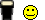1st one = 7

2nd one = -18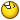IIRC, I was taught this stuff in the early to mid 60s. The way I was taught was that -5^2 == -25
This is weird. Is this old math then?I would have learned this in the early '80s (what grade do they do this in? 5? 6?) and I was taught that -5^2 == +25.

Maybe I'mbut here goe.......

Solve this problem:

-5² + 4 x 2³ =

-6² + 2 x 3² =

1. 572. 54Too bad the teacher sees it bryhamms way
The teacher is wrong.

Replies
13
Views
261
Replies
19
Views
626
Replies
248
Views
3K